# HSSlive: Plus One & Plus Two Notes & Solutions for Kerala State Board

## BSEB Class 10 Maths Chapter 8 Introduction to Trigonometry Ex 8.2 Textbook Solutions PDF: Download Bihar Board STD 10th Maths Chapter 8 Introduction to Trigonometry Ex 8.2 Book AnswersBSEB Class 10 Maths Chapter 8 Introduction to Trigonometry Ex 8.2 Textbook Solutions PDF: Download Bihar Board STD 10th Maths Chapter 8 Introduction to Trigonometry Ex 8.2 Book Answers

BSEB Class 10th Maths Chapter 8 Introduction to Trigonometry Ex 8.2 Textbooks Solutions and answers for students are now available in pdf format. Bihar Board Class 10th Maths Chapter 8 Introduction to Trigonometry Ex 8.2 Book answers and solutions are one of the most important study materials for any student. The Bihar Board Class 10th Maths Chapter 8 Introduction to Trigonometry Ex 8.2 books are published by the Bihar Board Publishers. These Bihar Board Class 10th Maths Chapter 8 Introduction to Trigonometry Ex 8.2 textbooks are prepared by a group of expert faculty members. Students can download these BSEB STD 10th Maths Chapter 8 Introduction to Trigonometry Ex 8.2 book solutions pdf online from this page.

## Bihar Board Class 10th Maths Chapter 8 Introduction to Trigonometry Ex 8.2 Books Solutions

 Board BSEB Materials Textbook Solutions/Guide Format DOC/PDF Class 10th Subject Maths Chapter 8 Introduction to Trigonometry Ex 8.2 Chapters All Provider Hsslive

## How to download Bihar Board Class 10th Maths Chapter 8 Introduction to Trigonometry Ex 8.2 Textbook Solutions Answers PDF Online?

2. Click on the Bihar Board Class 10th Maths Chapter 8 Introduction to Trigonometry Ex 8.2 Answers.
3. Look for your Bihar Board STD 10th Maths Chapter 8 Introduction to Trigonometry Ex 8.2 Textbooks PDF.
4. Now download or read the Bihar Board Class 10th Maths Chapter 8 Introduction to Trigonometry Ex 8.2 Textbook Solutions for PDF Free.

## BSEB Class 10th Maths Chapter 8 Introduction to Trigonometry Ex 8.2 Textbooks Solutions with Answer PDF Download

Find below the list of all BSEB Class 10th Maths Chapter 8 Introduction to Trigonometry Ex 8.2 Textbook Solutions for PDF’s for you to download and prepare for the upcoming exams:

## BSEB Bihar Board Class 10th Maths Solutions Chapter 8 Introduction to Trigonometry Ex 8.2

Question 1.
Evaluate :
(i) sin 60° cos 30° + sin 30° cos 60°
(ii) 2 tan2 45° + cos2 30° – sin2 60°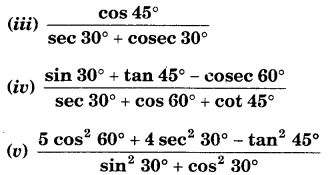Solution:
(i) sin 60° cos 30° + sin 30° cos 60°
= 3√2 x 3√2 + 12 x 12
= 34 + 14 = 3+14 = 44 = 1.

(ii) 2 tan² 45° + cos² 30° – sin² 60°
= 2(1)² + (3√2)2 – (3√2)2
= 2 + 34 – 34 = 2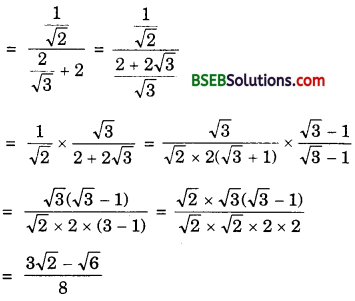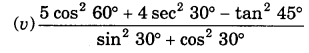Question 2.
Choose the correct option and justify:
(i) 2tan30∘1+tan230∘
(A) sin 60°
(B) cos 60°
(C) tan 60°
(D) sin 30°

(ii) 1−tan245∘1+tan245∘
(A) tan 90°
(B) 1
(C) sin 45°
(D) O

(iii) sin 2A = 2 sin A is true when A =
(A) 0°
(B) 30°
(C) 45°
(D) 60°

(iv) 2tan30∘1−tan230∘
(A) cos 60°
(B) sin 60°
(C) tan 60°
(D) none of these
Solution:
(i) (A)(ii) (D)
Because 1−tan245∘1+tan245∘ = 1−11+1 = 02 = 0

(iii) (A)
Because when A – 0°, sin 2A = sin 0° = 0
and, 2 sin A = 2 sin 0°= 2 x 0 = 0
or sin 2 A = 2 sin A, when A = 0°

(iv) (C)Question 3.
If tan (A + B) = 3‾√ and tan (A – B) = 13√; 0° < A + B ≤ 90°; A > B, find A and B.
Solution:
tan (A + B) = 3‾√ means tan (A + B) = tan 60°
So, A + B = 60° … (1)
tan (A – B) = 13√ means tan (A – B) = tan 30°
So, A – B = 30° … (2)
Solving (1) and (2), we get
A = 45° and B = 15°
Hence, A = 45° and B = 15°.

Question 4.
(i) sin (A + B) = sin A + sin B.
(ii) The value of sin θ increases as θ increases.
(iii) The value of cos θ increases as θ increases.
(iv) sin 0 = cos 0 for all values of 0.
(v) cot A is not defined for A = 0°.
Solution:
(i) False. Because
When A = 60° and B = 30°. Then,
sin (A + B) = sin (60° + 30°) = sin 90° = 1
and, sin A + sin B = sin 60° + sin 30°
= 3√2 + 12 = 3√+12
So, sin (A + B) ≠ sin A + sin B

(ii) True. Because, it is clear from the table below :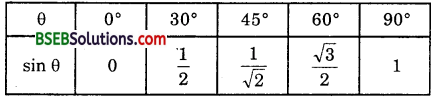that the value of sin θ increases as θ increases.

(iii) False. Because it is clear from the table below :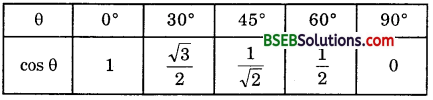that the value of cos θ decreases as θ increases.

(iv) False. Because it is only true for θ = 45°.
(sin 45° = 12√ = cos 45°)

(v) True. Because tan 0° = 0 and
cot 0° = 1tan0∘ = 10, i.e., not defined.

## Bihar Board Class 10th Maths Chapter 8 Introduction to Trigonometry Ex 8.2 Textbooks for Exam Preparations

Bihar Board Class 10th Maths Chapter 8 Introduction to Trigonometry Ex 8.2 Textbook Solutions can be of great help in your Bihar Board Class 10th Maths Chapter 8 Introduction to Trigonometry Ex 8.2 exam preparation. The BSEB STD 10th Maths Chapter 8 Introduction to Trigonometry Ex 8.2 Textbooks study material, used with the English medium textbooks, can help you complete the entire Class 10th Maths Chapter 8 Introduction to Trigonometry Ex 8.2 Books State Board syllabus with maximum efficiency.

## FAQs Regarding Bihar Board Class 10th Maths Chapter 8 Introduction to Trigonometry Ex 8.2 Textbook Solutions

#### How to get BSEB Class 10th Maths Chapter 8 Introduction to Trigonometry Ex 8.2 Textbook Answers??

Students can download the Bihar Board Class 10 Maths Chapter 8 Introduction to Trigonometry Ex 8.2 Answers PDF from the links provided above.

#### Can we get a Bihar Board Book PDF for all Classes?

Yes you can get Bihar Board Text Book PDF for all classes using the links provided in the above article.

## Important Terms

Bihar Board Class 10th Maths Chapter 8 Introduction to Trigonometry Ex 8.2, BSEB Class 10th Maths Chapter 8 Introduction to Trigonometry Ex 8.2 Textbooks, Bihar Board Class 10th Maths Chapter 8 Introduction to Trigonometry Ex 8.2, Bihar Board Class 10th Maths Chapter 8 Introduction to Trigonometry Ex 8.2 Textbook solutions, BSEB Class 10th Maths Chapter 8 Introduction to Trigonometry Ex 8.2 Textbooks Solutions, Bihar Board STD 10th Maths Chapter 8 Introduction to Trigonometry Ex 8.2, BSEB STD 10th Maths Chapter 8 Introduction to Trigonometry Ex 8.2 Textbooks, Bihar Board STD 10th Maths Chapter 8 Introduction to Trigonometry Ex 8.2, Bihar Board STD 10th Maths Chapter 8 Introduction to Trigonometry Ex 8.2 Textbook solutions, BSEB STD 10th Maths Chapter 8 Introduction to Trigonometry Ex 8.2 Textbooks Solutions,
Share: